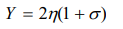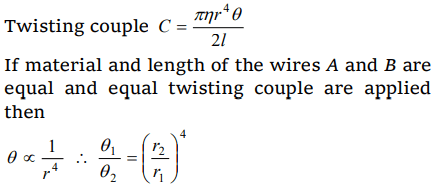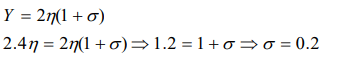## Mechanical Properties of Solids Questions and Answers Part-15

1. Which statement is true for a metal
a) $Y <\eta$
b) $Y =\eta$
c) $Y >\eta$
d) $Y <1\diagup\eta$

Explanation:2. Which of the following relations is true
a) $3Y = K\left(1-\sigma \right)$
b) $K =\frac{9\eta Y}{Y+\eta}$
c) $\sigma = \left(6K+\eta \right)Y$
d) $\sigma =\frac{0.5 Y -\eta}{\eta}$

Explanation:3.Two wires A and B of same length and of the same material have the respective radii $r_{1}$ and $r_{2}$ . Their one end is fixed with a rigid support, and at the other end equal twisting couple is applied. Then the ratio of the angle of twist at the end of A and the angle of twist at the end of B will be
a) $\frac{r_1^2}{r_2^2}$
b) $\frac{r_2^2}{r_1^2}$
c) $\frac{r_2^4}{r_1^4}$
d) $\frac{r_1^4}{r_2^4}$

Explanation:4. When a spiral spring is stretched by suspending a load on it, the strain produced is called
a) Shearing
b) Longitudinal
c) Volume
d) Transverse

Explanation: A small part of the spring bear tangential stress, causing straining strain.

5. The Young's modulus of the material of a wire is $6\times 10^{12}N\diagup m^{2}$  and there is no transverse strain in it, then its modulus of rigidity will be
a) $3\times 10^{12}N\diagup m^{2}$
b) $2\times 10^{12}N\diagup m^{2}$
c) $10^{12}N\diagup m^{2}$
d) None of the above

Explanation:6. If the Young's modulus of the material is 3 times its modulus of rigidity, then its volume elasticity will be
a) Zero
b) Infinity
c) $2\times 10^{10}N\diagup m^{2}$
d) $3\times 10^{10}N\diagup m^{2}$

Explanation:7. Modulus of rigidity of a liquid
a) Non zero constant
b) Infinite
c) Zero
d) Can not be predicted

Explanation: Zero

8. For a given material, the Young's modulus is 2.4 times that of rigidity modulus. Its Poisson's ratio is
a) 2.4
b) 1.2
c) 0.4
d) 0.2

Explanation:9. A cube of aluminium of sides 0.1 m is subjected to a shearing force of 100 N. The top face of the cube is displaced through 0.02 cm with respect to the bottom face. The shearing strain would be
a) 0.02
b) 0.1
c) 0.005
d) 0.002